# High School Math : Trigonometric Graphs

## Example Questions

### Example Question #1 : Understanding Period And Amplitude

What is the period of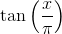?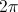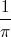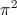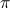Explanation:

The period for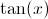is. However, if a number is multiplied by, you divide the periodby what is being multiplied by. Here,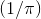is being multiplied by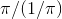equals### Example Question #2 : Understanding Period And Amplitude

What is the amplitude of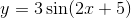?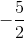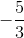Explanation:

The amplitude of a wave function likeis always going to be the coefficient of the function. In this case, that is.

### Example Question #3 : Understanding Period And Amplitude

What is the local maximum of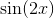between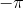and?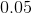Explanation:

The fastest way to solve this problem is to graph it and observe the answer. However, the other option is to think of this equation in terms of period.

When the coefficient of the variable increases, the frequency increases and the period decreases by that rate.

Since our equation is, our period will bethe normal period of a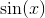wave. Since only the period is changing, the amplitude is not. Therefore the amplitude (the highest and lowest points) ofwill be the same as that of. The amplitude of a sine wave is, so the amplitude ofwill also be.

Therefore, our maximum will be.

### Example Question #8 : Period And Amplitude

Which of the given functions has the greatest amplitude?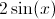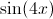The amplitude of a function is the amount by which the graph of the function travels above and below its midline. When graphing a sine function, the value of the amplitude is equivalent to the value of the coefficient of the sine. Similarly, the coefficient associated with the x-value is related to the function's period. The largest coefficient associated with the sine in the provided functions is 2; therefore the correct answer is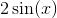.